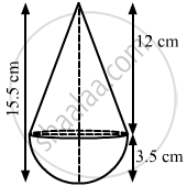Advertisement Remove all ads

# A Toy is in the Form of a Cone of Radius 3.5 Cm Mounted on a Hemisphere of Same Radius. the Total Height of the Toy is 15.5 Cm. Find the Total Surface Area of the Toy. - Mathematics

Sum

A toy is in the form of a cone of radius 3.5 cm mounted on a hemisphere of same radius. The total height of the toy is 15.5 cm. Find the total
surface area of the toy.

Advertisement Remove all ads

#### SolutionWe have,

Radius of the hemisphere = Radius of the cone = r = 3.5 cm and

Height of the cone = 15.5 - 3.5 = 12 cm

Also,

The slant height of the cone, "l" = sqrt("h"^2 +"r"^2)'

= sqrt(12^2+3.5^2)

=sqrt(144+12.25)

=sqrt(156.25)

=12.5  cm

Now,

Total surface area of the toy = CSA of cone + CSA of hemisphere

=pi"rl" + 2pi"r"^2

=pir("l"+2"r")

=22/7xx3.5xx(12.5+2xx3.5)

=11xx(12.5 + 7)

=11xx19.5

=214.5 cm

So, the total surface area of the toy is 214.5 cm2.

Disclaimer: The answer given in the textbook is incorrect. The same has been corrected above.

Is there an error in this question or solution?
Advertisement Remove all ads

#### APPEARS IN

RS Aggarwal Secondary School Class 10 Maths
Chapter 19 Volume and Surface Area of Solids
Exercise | Q 35 | Page 916
Advertisement Remove all ads
Advertisement Remove all ads
Share
Notifications

View all notifications

Forgot password?